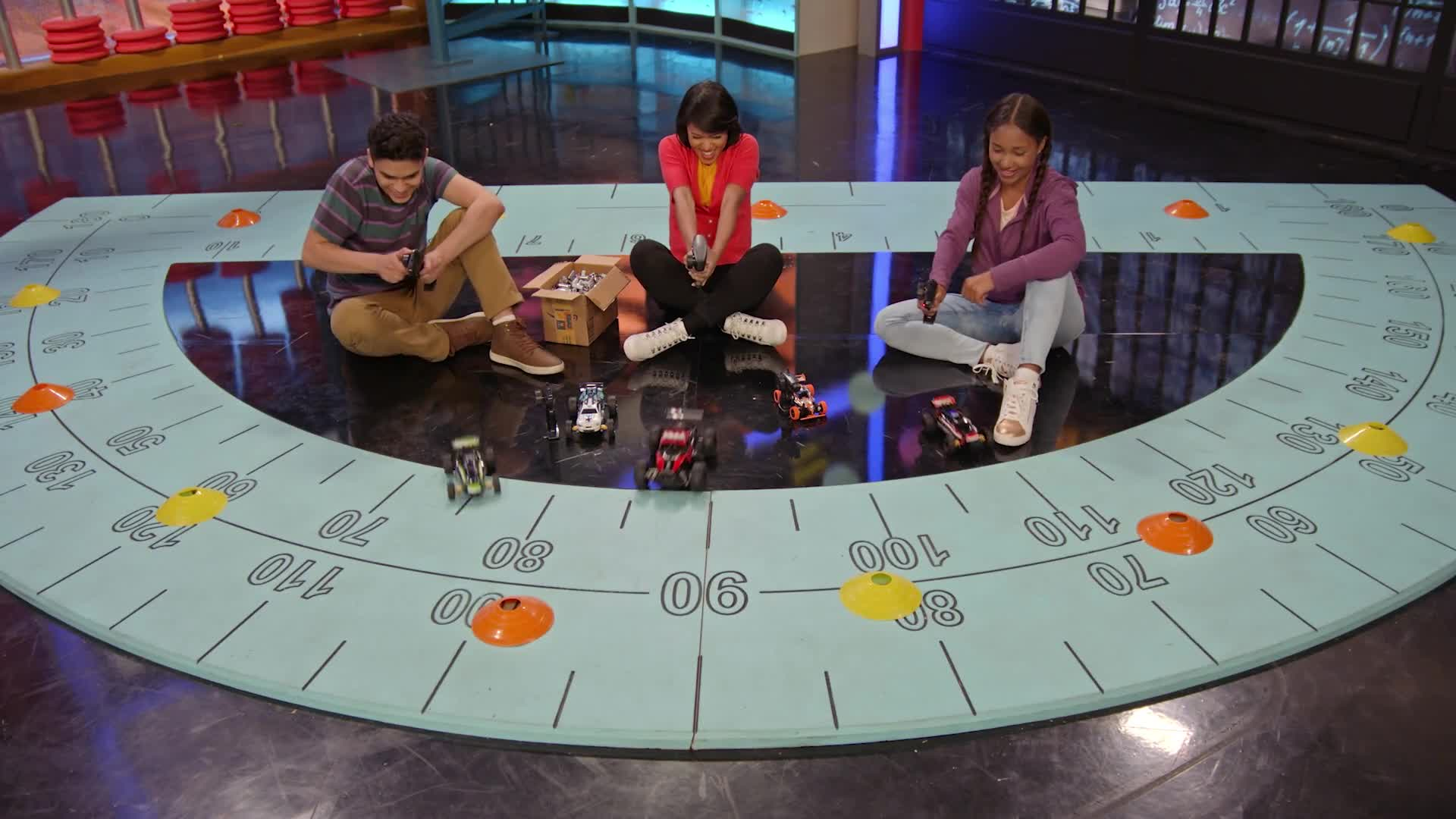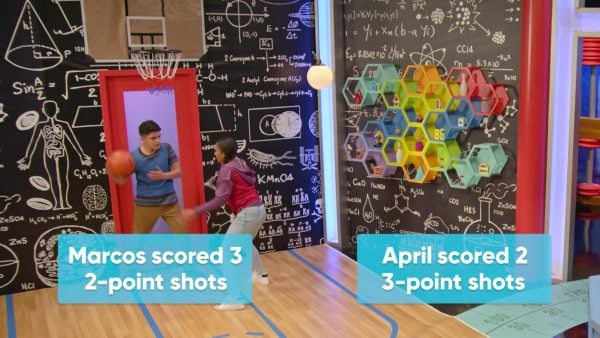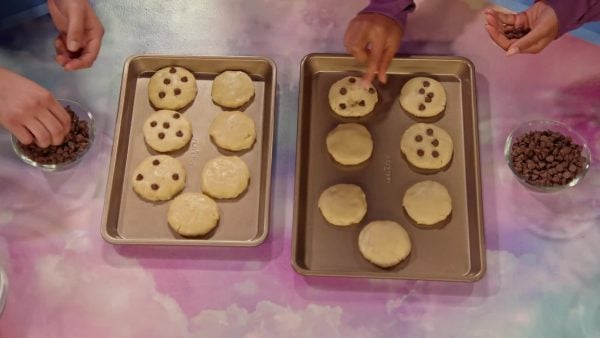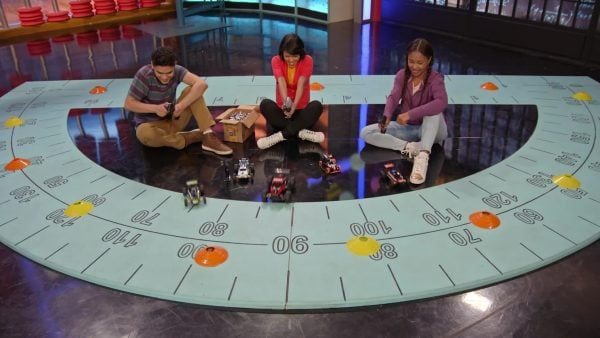Multiplication Properties Math Video for Kids | 3rd, 4th & 5th Grade
1%
It was processed successfully!WHAT ARE MULTIPLICATION PROPERTIES: COMMUTATIVE, ASSOCIATIVE, AND DISTRIBUTIVE?

Properties of multiplication are special facts about multiplication that you can use to find products. These properties can help you break down seemingly difficult multiplication problems into simpler ones.

To better understand multiplication properties…

WHAT ARE MULTIPLICATION PROPERTIES: COMMUTATIVE, ASSOCIATIVE, AND DISTRIBUTIVE?. Properties of multiplication are special facts about multiplication that you can use to find products. These properties can help you break down seemingly difficult multiplication problems into simpler ones. To better understand multiplication properties…

## LET’S BREAK IT DOWN!April and Marcos are playing basketball. April scored two 3-point shots and Marcos scored three 2-point shots. Who scored more points? April’s score can be expressed as 2 × 3, which equals 6. Marcos’ score can be expressed as 3 × 2, which also equals 6. Their scores are the same, and we have discovered the commutative property of multiplication: The order in which we multiply two factors does not change the product. Try this one yourself: You have 3 boxes that each contain 5 cookies and your friend has 5 boxes that each contain 3 cookies. Show that you and your friend have the same number of cookies.

Basketball April and Marcos are playing basketball. April scored two 3-point shots and Marcos scored three 2-point shots. Who scored more points? April’s score can be expressed as 2 × 3, which equals 6. Marcos’ score can be expressed as 3 × 2, which also equals 6. Their scores are the same, and we have discovered the commutative property of multiplication: The order in which we multiply two factors does not change the product. Try this one yourself: You have 3 boxes that each contain 5 cookies and your friend has 5 boxes that each contain 3 cookies. Show that you and your friend have the same number of cookies.

### PostcardsAdesina, April, and Marcos have postcards arranged in a 12 by 6 grid. How many postcards do they have? The distributive property will help to solve this multiplication problem more easily than multiplying 12 by 6. is the grid can be divided into two smaller rectangles, so that one rectangle measures 10 by 6 postcards and the other measures 2 by 6. These calculations are easier than the original multiplication, and once we have the products, we can add them together to find the total number of postcards. This expression can be written as 10 × 6 + 2 × 6, and we can use parentheses to make the expression easier to read: (10 × 6) + (2 × 6) = 60 + 12 = 72. Since 12 × 6 also equals 72, we have shown that multiplying two factors gives us the same answer as splitting one of the factors, multiplying both parts by the other factor, and adding the products together. Try this one yourself: Use the distributive property to find an easier way to find the number of postcards in a 19 by 8 grid.

Postcards Adesina, April, and Marcos have postcards arranged in a 12 by 6 grid. How many postcards do they have? The distributive property will help to solve this multiplication problem more easily than multiplying 12 by 6. is the grid can be divided into two smaller rectangles, so that one rectangle measures 10 by 6 postcards and the other measures 2 by 6. These calculations are easier than the original multiplication, and once we have the products, we can add them together to find the total number of postcards. This expression can be written as 10 × 6 + 2 × 6, and we can use parentheses to make the expression easier to read: (10 × 6) + (2 × 6) = 60 + 12 = 72. Since 12 × 6 also equals 72, we have shown that multiplying two factors gives us the same answer as splitting one of the factors, multiplying both parts by the other factor, and adding the products together. Try this one yourself: Use the distributive property to find an easier way to find the number of postcards in a 19 by 8 grid.

### Chocolate ChipsApril and Marcos are making chocolate chip cookies. Each of them has 7 cookies and each cookie needs 5 chocolate chips. How many chocolate chips do they need in total? To find the total, we multiply 7 (the number of cookies each person has) by 5 (the number of chocolate chips each cookie gets) by 2 (the number of cookie makers): 7 × 5 × 2. Does it make a difference if we calculate 7 × 5 before multiplying by 2, or calculate 5 × 2 first before multiplying by 7? Let's try both methods. We use parentheses to indicate the part of the expression that we solve first. The first way, we write (7 × 5) × 2 = 35 × 2 = 70. The second way, we write 7 × (5 × 2) = 7 × 10 = 70 as well. We have just shown that the grouping of factors does not change the product, the associative property of multiplication. The second method was a little bit easier to solve, because multiplying by 10 is simple. Try this one yourself: You are baking 5 boxes of 7 banana chocolate chip muffins. Each muffin needs 8 chocolate chips. Use the associative property to find the simplest way to find the total number of chocolate chips needed.

Chocolate Chips April and Marcos are making chocolate chip cookies. Each of them has 7 cookies and each cookie needs 5 chocolate chips. How many chocolate chips do they need in total? To find the total, we multiply 7 (the number of cookies each person has) by 5 (the number of chocolate chips each cookie gets) by 2 (the number of cookie makers): 7 × 5 × 2. Does it make a difference if we calculate 7 × 5 before multiplying by 2, or calculate 5 × 2 first before multiplying by 7? Let's try both methods. We use parentheses to indicate the part of the expression that we solve first. The first way, we write (7 × 5) × 2 = 35 × 2 = 70. The second way, we write 7 × (5 × 2) = 7 × 10 = 70 as well. We have just shown that the grouping of factors does not change the product, the associative property of multiplication. The second method was a little bit easier to solve, because multiplying by 10 is simple. Try this one yourself: You are baking 5 boxes of 7 banana chocolate chip muffins. Each muffin needs 8 chocolate chips. Use the associative property to find the simplest way to find the total number of chocolate chips needed.

### Introduction Revisited: RacetrackApril and Marcos use remote controls to race cars. There are 4 teams, each team has 12 cars, and each car needs 5 batteries. April suggests that the total number of batteries they need can be found using the expression 4 × 12 × 5, and Marcos suggests using the expression 12 × 4 × 5. Who is right? Now that we are familiar with the commutative, associative, and distributive properties, we know that they are both correct. Because of the commutative property, we know that we can arrange the numbers to be multiplied in any order. The associative property allows us to choose to multiply 4 × 5 first to get 20. Then we multiply 12 × 20. Using the distributive property, we can split 12: 12 = 10 + 2. So we multiply 10 × 20 and 2 × 20 and add the products together: 200 + 40 = 420.

Introduction Revisited: Racetrack April and Marcos use remote controls to race cars. There are 4 teams, each team has 12 cars, and each car needs 5 batteries. April suggests that the total number of batteries they need can be found using the expression 4 × 12 × 5, and Marcos suggests using the expression 12 × 4 × 5. Who is right? Now that we are familiar with the commutative, associative, and distributive properties, we know that they are both correct. Because of the commutative property, we know that we can arrange the numbers to be multiplied in any order. The associative property allows us to choose to multiply 4 × 5 first to get 20. Then we multiply 12 × 20. Using the distributive property, we can split 12: 12 = 10 + 2. So we multiply 10 × 20 and 2 × 20 and add the products together: 200 + 40 = 420.

## MULTIPLICATION PROPERTIES VOCABULARY

Product
The result when numbers are multiplied together.
Factor
A number that divides another number.
Parentheses
Symbols ( ) that show us which part of an expression to solve first.
Commutative
The property of multiplication stating that the order in which we multiply factors does not change the product.
Associative
The property of multiplication stating that different ways of grouping factors does not change the product.
Distributive
The property of multiplication stating that multiplying two factors gives the same answer as splitting one of the factors, multiplying both parts by the other factor, and adding the products together.

## MULTIPLICATION PROPERTIES DISCUSSION QUESTIONS

### State the commutative property in your own words.

When we multiply, the order in which we multiply does not change the product.

### What is the name of the property that says that multiplying two factors is the same as splitting one of the factors into two parts, multiplying both parts by the other factor, and adding the products together?

The distributive property.

### State the associative property in your own words.

When we multiply, we can group the factors in any order and the product will be the same.

### We can use the distributive property to write 5 × 23 as (5 × 4) + (5 × 19). Does this make it easier to find the product? Can you think of a better way to split 23?

No, this expression is not easier. A better way is to split 23 into 20 and 3: (5 × 20) + (5 × 3).

### Put parentheses around the factors that you will multiply first to make it easier to solve: 9 × 8 × 5. Which property allows you to do that?

9 × (8 × 5); the associative property.
X

## Success

We’ve sent you an email with instructions how to reset your password.
Ok

## Choose Your Free Trial Period### 7 DaysContinue to Lessons### 90 DaysGet 90 days free by inviting other teachers to try it too.

Share with Teachers

## Get 90 Days Free

### By inviting 4 other teachers to try it too.

4 required

Skip, I will use a 7 days free trial

## Thank You!

Enjoy your free 90 days trial